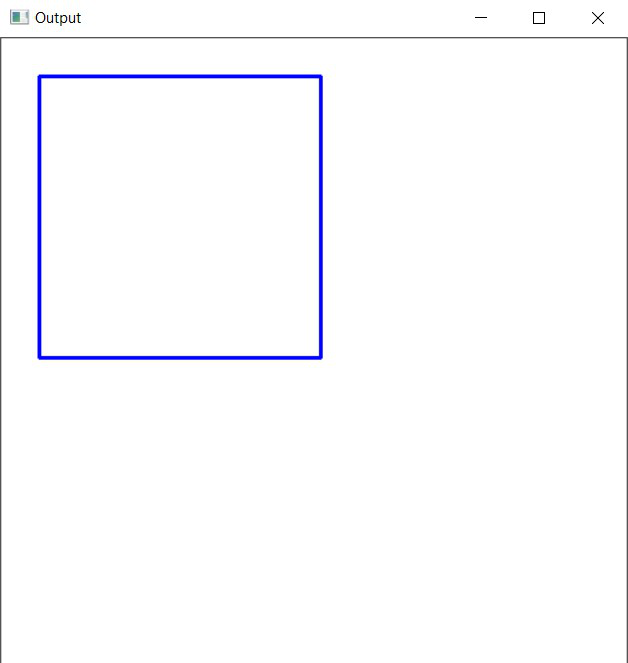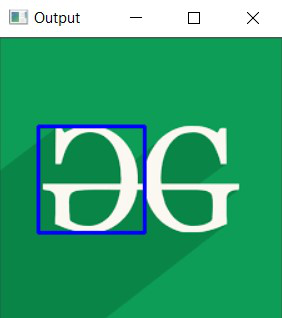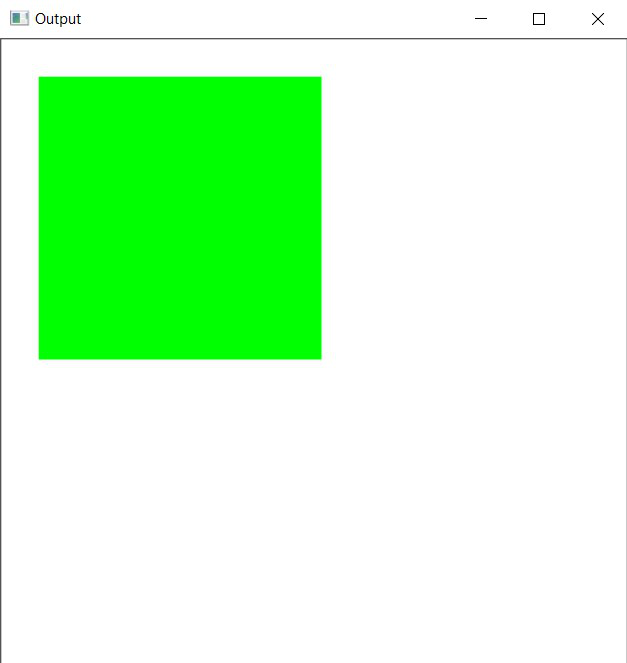# Draw an rectangle using OpenCV in C++

• Last Updated : 19 Mar, 2021

In this article, the task is to draw an rectangle using OpenCV in C++. The rectangle() function from OpenCV C++ library will be used.

Syntax:

rectangle( img, pt1, pt2, color, thickness, line Type, shift)

Parameters:

• image: It is the image on which the rectangle is to be drawn.
• start(pt1): It is the top left corner of the rectangle represented as the tuple of two coordinates i.e., (x-coordinate, y-coordinate).
• end(pt2): It is the bottom right corner of the rectangle represented as the tuple of two coordinates i.e., (x-coordinate, y-coordinate).
• color: It is the color of the borderline of the rectangle to be drawn. A tuple representing 3 colors (B, G, R) i.e., (Blue, Green, Red).
• thickness: It is the thickness of the rectangle borderline in px. The thickness of -1 px will fill the rectangle shape by the specified color.
• lineType: Type of the line. There are 3 types of line:
• LINE_4: Line was drawn using 4 connected Bresenham algorithm.
• LINE_8: Line was drawn using 8 connected Bresenham algorithm.
• LINE_AA: It draws Anti-aliased lines formed by using a Gaussian filter.
• shift: The number of fractional bits in the point coordinates.

Return Value: It returns an image.

Program 1:

Below is the C++ program demonstrating how to draw a rectangle over a self-formed background image:

## C++

 `// C++ program to demonstrate rectangle ` `// over a self-formed background image ` ` `  `#include ` `#include ` ` `  `// Drawing shapes ` `#include ` ` `  `#include ` `using` `namespace` `cv; ` `using` `namespace` `std; ` ` `  `// Driver Code ` `int` `main(``int` `argc, ``char``** argv) ` `{ ` `    ``// Creating a blank image with ` `    ``// white background ` `    ``Mat image(500, 500, CV_8UC3, ` `              ``Scalar(255, 255, 255)); ` ` `  `    ``// Check if the image is created ` `    ``// successfully or not ` `    ``if` `(!image.data) { ` `        ``std::cout << ``"Could not open or "` `                  ``<< ``"find the image\n"``; ` ` `  `        ``return` `0; ` `    ``} ` ` `  `    ``// Top Left Corner ` `    ``Point p1(30, 30); ` ` `  `    ``// Bottom Right Corner ` `    ``Point p2(255, 255); ` ` `  `    ``int` `thickness = 2; ` ` `  `    ``// Drawing the Rectangle ` `    ``rectangle(image, p1, p2, ` `              ``Scalar(255, 0, 0), ` `              ``thickness, LINE_8); ` ` `  `    ``// Show our image inside a window ` `    ``imshow(``"Output"``, image); ` `    ``waitKey(0); ` ` `  `    ``return` `0; ` `} `

Output:Program 2:

Below is the C++ program demonstrating how to draw a rectangle over the image of the GFG Logo:

## C++

 `// C++ program to demonstrate rectangle ` `// over a loaded image of GFG logo ` ` `  `#include ` `#include ` ` `  `// Drawing shapes ` `#include ` ` `  `#include ` `using` `namespace` `cv; ` `using` `namespace` `std; ` ` `  `// Driver Code ` `int` `main(``int` `argc, ``char``** argv) ` `{ ` `    ``// Reading the Image ` `    ``Mat image = imread(``"C:/Users/harsh/Downloads/geeks.png"``, ` `                       ``IMREAD_COLOR); ` ` `  `    ``// Check if the image is created ` `    ``// successfully or not ` `    ``if` `(!image.data) { ` `        ``std::cout << ``"Could not open or "` `                  ``<< ``"find the image\n"``; ` `        ``return` `0; ` `    ``} ` ` `  `    ``// Top Left Coordinates ` `    ``Point p1(30, 70); ` ` `  `    ``// Bottom Right Coordinates ` `    ``Point p2(115, 155); ` ` `  `    ``int` `thickness = 2; ` ` `  `    ``// Drawing the Rectangle ` `    ``rectangle(image, p1, p2, ` `              ``Scalar(255, 0, 0), ` `              ``thickness, LINE_8); ` ` `  `    ``// Show our image inside a window ` `    ``imshow(``"Output"``, image); ` `    ``waitKey(0); ` ` `  `    ``return` `0; ` `}`

Output:Program 3:

Below is the C++ program demonstrating how to draw a rectangle filled with color:

## C++

 `// C++ program to demonstrate rectangle ` `// filled with any color ` ` `  `#include ` `#include ` ` `  `// Drawing shapes ` `#include ` ` `  `#include ` `using` `namespace` `cv; ` `using` `namespace` `std; ` ` `  `// Driver Code ` `int` `main(``int` `argc, ``char``** argv) ` `{ ` `    ``// Creating a blank image with ` `    ``// white background ` `    ``Mat image(500, 500, CV_8UC3, ` `              ``Scalar(255, 255, 255)); ` ` `  `    ``// Check if the image is created ` `    ``// successfully or not ` `    ``if` `(!image.data) { ` `        ``std::cout << ``"Could not open or "` `                  ``<< ``"find the image\n"``; ` ` `  `        ``return` `0; ` `    ``} ` ` `  `    ``// Top Left Corner ` `    ``Point p1(30, 30); ` ` `  `    ``// Bottom Right Corner ` `    ``Point p2(255, 255); ` ` `  `    ``int` `thickness = -1; ` ` `  `    ``// Drawing the Rectangle ` `    ``rectangle(image, p1, p2, ` `              ``Scalar(0, 255, 0), ` `              ``thickness, LINE_8); ` ` `  `    ``// Show our image inside a window ` `    ``imshow(``"Output"``, image); ` `    ``waitKey(0); ` ` `  `    ``return` `0; ` `} `

Output:My Personal Notes arrow_drop_up
Recommended Articles
Page :